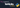# TIL: Bind arguments to dynamically generated lambdas in python

This past week I had a really weird bug in my kedro pipeline. For some reason data running through my pipeline was coming out completely made no sense, but if I manually request raw data outside of the pipeline it matched expectations.

NOTE While this story is about a kedro pipeline, it can be applied anywhere closures are put into an iterable.

##After a few days of looking at it off and on, I pinpointed that it was all the way down in the raw layer. Right as data is coming off of the database. For this I already had existing `sql` files stored and a `read_sql` function to get the data so I opted to just set up the pipeline to utilize the existing code as much as possible, leaning on the kedro framework a bit less.

I have dynamically created lists of pipeline nodes many times in the past, but typically I take data from kedro input and use it in the lambda. I prefer the simplicity of using lambdas over `functools.partial`. It typically looks something like this.

``````# 👍  I do this all the time
from kedro.pipeline import node
from my_generic_project_lib import clean

datasets_to_clean = ['sales', 'production', 'inventory']
nodes = []
for dataset in datasets_to_clean:
nodes.append(
node(
func=lambda x: clean(x)
inputs = f'raw_{dataset}'
outputs=f'int_{dataset}'
tags=['int', dataset]
name=f'create_int_{dataset}'
)
)``````

What was different this time is that I needed to pass in the name of the dataset to my read_sql function, not the data loaded in the framework.

``````# ❌ This does not work
from kedro.pipeline import node

nodes = []
for dataset in datasets_to_clean:
nodes.append(
node(
func=lambda: read_sql(dataset) # 💥 The major issue
inputs = f'dummy'
outputs=f'int_{dataset}'
tags=['int', dataset]
name=f'create_int_{dataset}'
)
)``````

##As I am still oblivious to what has happened I pop in a `breakpoint()` and quickly see that during the first run the dataset passed into `read_sql` was `'inventory'`, in fact, every single one was `'inventory'`. The lambda is just using the latest value of dataset from outside and has no `local` `dataset` attached to it.

##``````# 👍 Much Better
from kedro.pipeline import node

nodes = []
for dataset in datasets_to_clean:
nodes.append(
node(
func=lambda dataset=dataset: read_sql(dataset) # dataset is now bound to the lambda ✨
inputs = f'dummy'
outputs=f'int_{dataset}'
tags=['int', dataset]
name=f'create_int_{dataset}'
)
)``````

##I made a slightly more simple example so that you can try it and play with it yourself, edit it, share it with your friends, laugh at my mistake, whatever you like.

👀 see an issue, edit this post on GitHub

If you found value in this post
and want to send a tip.Check out my otherblogs